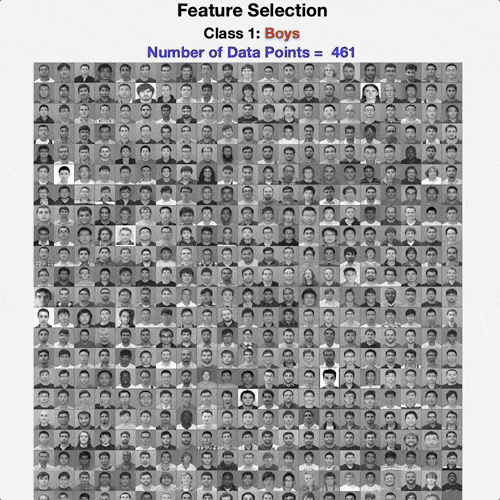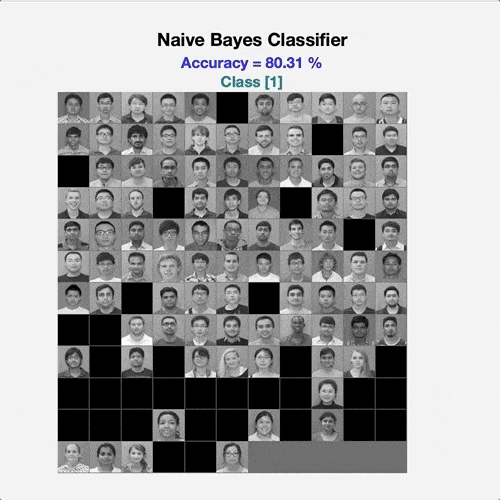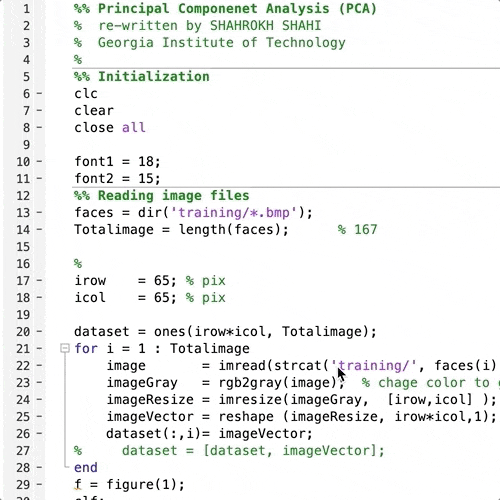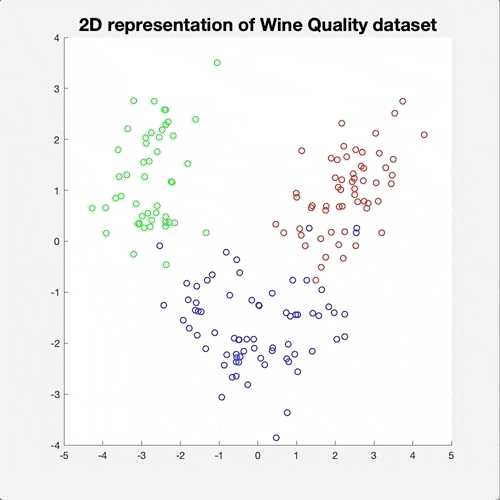#### Feature Selection based on Information Theory

Finding the most informative dimensions (pixels) of face images, given a dataset of two classes of males and females images. The most informative pixels are demonstrated by a heatmap of informativeness scoresFeature selection program to pick the most informative pixels

#### Binary Classification: Naive Bayesian Classifier, K-Nearest Neighbors (KNN), and Logistic Regression

Employing three different classification algorithms to classify images into two male and female classes. It is shown that logistic regression works slightly better than the other two approachesBinary classification approaches

#### Principal Component Analysis (PCA)

Conducting Principal Component Analysis (PCA) on a dataset of face images to find the principal directions. The analysis shows that by keeping the top 50 dimensions, we can pretty much capture all the information in the dataset.Principal component analysis

#### Density Estimation with KDE and GMM

«p align=”justify”> First, running PCA on 13-dimensional “Wine Quality” dataset to reduce the dimension to two and visualize the multi-modal behaviour. Then, using the Expectation-Maximization (EM) algorithm to fit a Gaussian Mixture Model (GMM) to the data and comparing the results with Kernel Density Estimation (KDE) and Histogram </p>Density estimation approaches; KDE and GMM

#### K-Means Clustering

A Simple K-Means Clustering TestK-means clustering